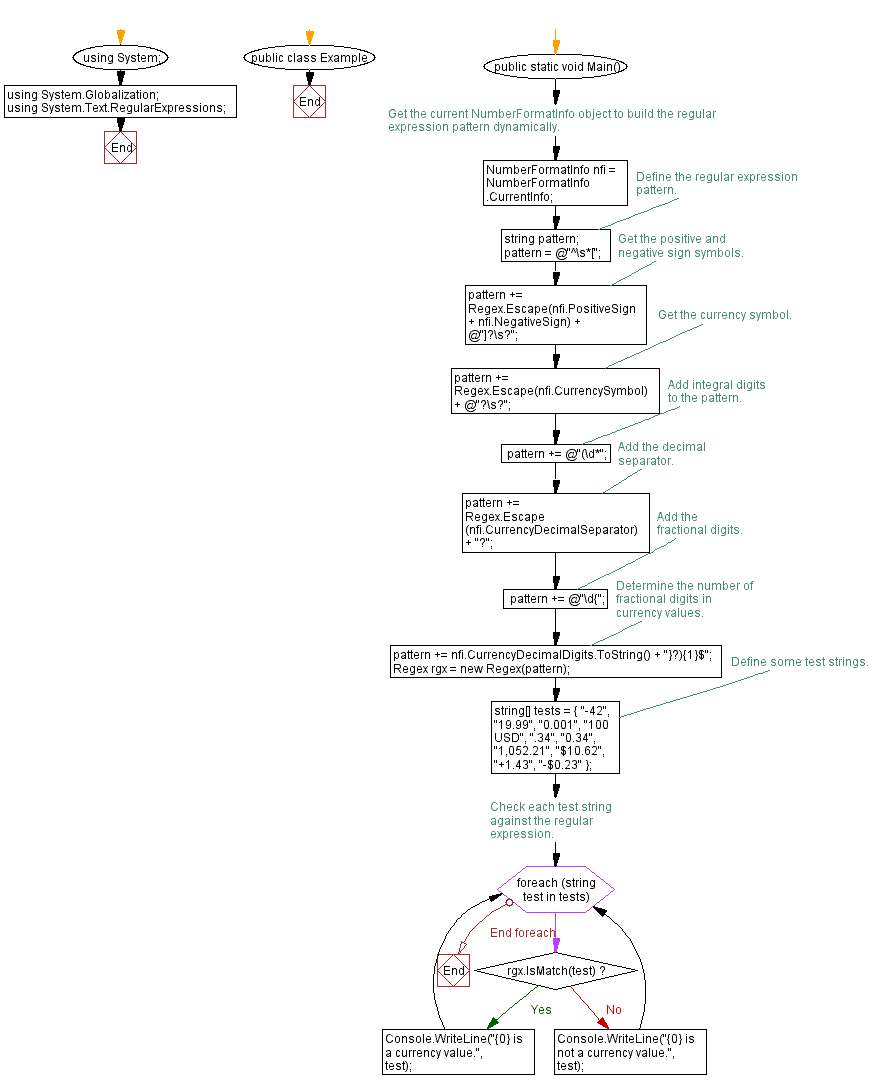﻿ C# - Check whether a string represents a currency value# C# Sharp Exercises: Check whether a string represents a currency value

## C# Sharp Regular Expression: Exercise-7 with Solution

Write a C# Sharp program to check whether a given string either represents a currency value or has the correct format to represent a currency value.

Sample Solution:

C# Sharp Code:

``````using System;
using System.Globalization;
using System.Text.RegularExpressions;

public class Example
{
public static void Main()
{
// Get the current NumberFormatInfo object to build the regular
// expression pattern dynamically.
NumberFormatInfo nfi = NumberFormatInfo.CurrentInfo;

// Define the regular expression pattern.
string pattern;
pattern = @"^\s*[";
// Get the positive and negative sign symbols.
pattern += Regex.Escape(nfi.PositiveSign + nfi.NegativeSign) + @"]?\s?";
// Get the currency symbol.
pattern += Regex.Escape(nfi.CurrencySymbol) + @"?\s?";
// Add integral digits to the pattern.
pattern += @"(\d*";
// Add the decimal separator.
pattern += Regex.Escape(nfi.CurrencyDecimalSeparator) + "?";
// Add the fractional digits.
pattern += @"\d{";
// Determine the number of fractional digits in currency values.
pattern += nfi.CurrencyDecimalDigits.ToString() + "}?){1}\$";

Regex rgx = new Regex(pattern);

// Define some test strings.
string[] tests = { "-42", "19.99", "0.001", "100 USD",
".34", "0.34", "1,052.21", "\$10.62",
"+1.43", "-\$0.23" };

// Check each test string against the regular expression.
foreach (string test in tests)
{
if (rgx.IsMatch(test))
Console.WriteLine("{0} is a currency value.", test);
else
Console.WriteLine("{0} is not a currency value.", test);
}
}
}
```
```

Sample Output:

```-42 is a currency value.
19.99 is a currency value.
0.001 is not a currency value.
100 USD is not a currency value.
.34 is a currency value.
0.34 is a currency value.
1,052.21 is not a currency value.
\$10.62 is a currency value.
+1.43 is a currency value.
-\$0.23 is a currency value.
```

Flowchart:C# Sharp Code Editor:

Improve this sample solution and post your code through Disqus

Previous C# Sharp Exercise: Check for repeated occurrences of words in a string.
Next C# Sharp Exercise: Remove special characters from a given text.

What is the difficulty level of this exercise?

Test your Programming skills with w3resource's quiz.

﻿